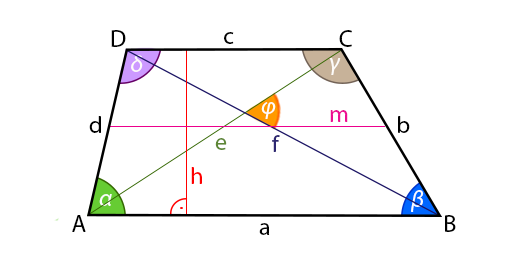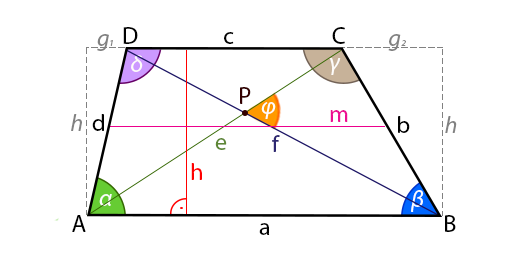Choose language

PL, EN, ES, DE, FR, RU

# Trapezoid calculator - diagonals, area, perimeter, sides lenght

Trapezium (trapezoid) calculator will help you calculate the long diagonal of the trapezium, the short diagonal of the trapezium, the side length, height, area and perimeter of the trapezium.

## First diagonal of the trapezoid - calculator

### First diagonal of the trapezoid from the sides (a) and (b) and the angle β

$$e=\sqrt{a^2+b^2-2ab\cdot \cos(\beta)}$$

### First diagonal of the trapezoid from the sides (c) and (d) and the angle δ

$$e=\sqrt{c^2+d^2-2cd\cdot \cos(\delta)}$$

### First diagonal of the trapezoid from sides (a)(b)(c)(d)

$$e= \sqrt{\frac{ac^2 - a^2c - ad^2 + b^2c}{c-a}}$$## Second diagonal of the trapezoid - calculator

### Second diagonal of the trapezoid from the sides (a) (d) and the angle α

$$f=\sqrt{a^2+d^2-2ad\cdot \cos(\alpha)}$$

### Second diagonal of the trapezoid from the sides (b) (c) and the angle γ

$$f=\sqrt{b^2+c^2-2bc\cdot \cos(\gamma)}$$

### Second diagonal of the trapezoid from the sides (a)(b)(c)(d)

$$f= \sqrt{\frac{ac^2 - a^2c - ab^2 + cd^2}{c-a}}$$## Height of the trapezoid - calculator

### Height of the trapezoid from the sides (a)(c) and area

$$h=\frac{2S}{a+c}$$

### Height of the trapezoid from the sides (b)(d) and angle

$$h=b \cdot \sin \beta = b \cdot \sin \gamma$$ $$= d \cdot \sin \alpha = d \cdot \sin \delta$$

### Height of the trapezoid from the sides

$$h= \frac{\sqrt{i \cdot j \cdot k \cdot l}}{2|c-a|}$$ where: $$i=-a+b+c+d,$$$$j=a+b-c+d,$$$$k=a+b-c-d,$$$$l=a-b-c+d.$$## Area of the trapezoid - calculator

### Area of the trapezoid from sides (a) and (c) and the height (h)

$$S={\frac {a+c}{2}}\cdot h$$

### Trapezoidal area from sides and angle (α) or angle(β)

$$S=\frac{1}{2}\cdot\left(a+c\right)\cdot d\cdot \sin\beta$$$$S=\frac{1}{2}\cdot\left(a+c\right)\cdot b\cdot \sin\beta$$

### Area of the trapezoid from the sides (a)(b)(c)(d)

$$S=\frac{a+c}{4|c-a|}\sqrt{i \cdot j \cdot k \cdot l}.$$ where: $$i=-a+b+c+d,$$$$j=a+b-c+d,$$$$k=a+b-c-d,$$$$l=a-b-c+d.$$## Perimeter of the trapezoid - calculator

### Perimeter of the trapezoid from the sides

$$L = a + b + c + d$$

### Perimeter of the trapezoid from bases, heights and angles (α) and (β)

$$L = a+c+h \cdot(\frac{1}{\sin(\alpha)}+\frac{1}{\sin(\beta)})$$## Sides of the trapezoid - calculator

### Side (a) of the trapezoid from the sides (b)(c)(d) and height (h)

$$a=c+\sqrt{d^2-h^2}+\sqrt{b^2-h^2}$$

### Side (b) of the trapezoid from the sides (a)(c)(d) and height (h)

$$b=\sqrt{h^2-(-a+c+\sqrt{d^2-h^2})^2}$$

### Side (c) of the trapezoid from sides (a)(b)(d) and height (h)

$$c=a-\sqrt{d^2-h^2}-\sqrt{b^2-h^2}$$

### Side (d) of the trapezoid from sides (a)(b)(c) and height (h)

$$d=\sqrt{h^2+(a-c-\sqrt{b^2-h^2})^2}$$## Midline (median , midsegment) of the trapezoid - calculator

### Midline (median , midsegment) of the trapezoid

$$m=\frac {a+c}{2}$$Trapezoid - information

Trapezoid
– a quadrilateral having at least one pair of parallel sides; The (selected) pair of parallel sides are called the bases, the remaining sides are called the legs, the distance between the bases is called the height of the trapezoid. Some colloquial definitions define a trapezoid as a quadrilateral with only one pair of parallel sides and, accordingly, the parallelogram is not a trapezoid. The sum of the angles on the same legs is 180°. If P is the intersection of the diagonals, then it's triangles ΔADP and ΔBCP have equal fields, ΔABP and ΔCDP are similar. Triangles ΔABC and ΔABD have a common base and equal height, and therefore equal fields. Triangles ΔBCP and ΔADP they arise from them by "subtracting" the triangle ΔABP.Trapezoid has the following properties:
1. Trapezoid is a convex figure.
2. The sum of the measures of all interior angles is 2Π (360 °), and the sum of the measures of two adjacent internal angles lying on the same leg is Π, $$\alpha + \delta = 180°$$$$\beta + \gamma = 180°$$
3. Formula for the first diagonal of the trapezoid from the sides (a) and (b) and angle β
4. $$e=\sqrt{a^2+b^2-2ab\cdot \cos(\beta)}$$
5. Formula for the first diagonal of the trapezoid from sides (c) i (d) and angle δ
6. $$e=\sqrt{c^2+d^2-2cd\cdot \cos(\delta)}$$
7. Formula for the first diagonal of the trapezoid from sides (a)(b)(c)(d)
8. $$e= \sqrt{\frac{ac^2 - a^2c - ad^2 + b^2c}{c-a}}$$
9. Formula for the second diagonal of the trapezoid from sides (a) i (d) and angle α
10. $$f=\sqrt{a^2+d^2-2ad\cdot \cos(\alpha)}$$
11. Formula for the second diagonal of the trapezoid from sides (b) i (c) and angle γ
12. $$f=\sqrt{b^2+c^2-2bc\cdot \cos(\gamma)}$$
13. Formula for the second diagonal of the trapezoid from sides (a)(b)(c)(d)
14. $$f= \sqrt{\frac{ac^2 - a^2c - ab^2 + cd^2}{c-a}}$$
15. Formula for height of the trapezoid from the sides (a)(c) and area
16. $$h=\frac{2S}{a+c}$$
17. Formula for height of the trapezoid from the sides (b)(d) and angle
18. $$h=b \cdot \sin \beta = b \cdot \sin \gamma$$ $$= d \cdot \sin \alpha = d \cdot \sin \delta$$
19. Formula for height of the trapezoid from the sides
20. $$h= \frac{\sqrt{i \cdot j \cdot k \cdot l}}{2|c-a|}$$ where: $$i=-a+b+c+d,$$$$j=a+b-c+d,$$$$k=a+b-c-d,$$$$l=a-b-c+d.$$
21. Formula for area of the trapezoid from sides (a) and (b) and the height (h)
22. $$S={\frac {a+c}{2}}\cdot h$$
23. Formula for trapezoidal area from sides and angle (α) or angle(β)
24. $$S=\frac{1}{2}\cdot\left(a+c\right)\cdot d\cdot \sin\beta$$$$S=\frac{1}{2}\cdot\left(a+c\right)\cdot b\cdot \sin\beta$$
25. Formula for area of the trapezoid from the sides (a)(b)(c)(d)
26. $$S=\frac{a+c}{4|c-a|}\sqrt{i \cdot j \cdot k \cdot l}.$$ where: $$i=-a+b+c+d,$$$$j=a+b-c+d,$$$$k=a+b-c-d,$$$$l=a-b-c+d.$$
27. Wzór na Perimeter of the trapezoid from the sides
28. $$L = a + b + c + d$$
29. Formula for perimeter of a trapezoid from bases, heights and angles (α) and (β)
30. $$L = a+c+h*(\frac{1}{\sin(\alpha)}+\frac{1}{\sin(\beta)})$$
31. Formula for lenght side (a) from sides (b)(c)(d) and height (h)
32. $$a=c+\sqrt{d^2-h^2}+\sqrt{b^2-h^2}$$
33. Formula for lenght leg (b) from sides (a)(c)(d) and height (h)
34. $$b=\sqrt{h^2-(-a+c+\sqrt{d^2-h^2})^2}$$
35. Formula for lenght side (c) from sides (a)(b)(d) and height (h)
36. $$c=a-\sqrt{d^2-h^2}-\sqrt{b^2-h^2}$$
37. Formula for lenght leg (d) from sides (a)(b)(c) and height (h)
38. $$d=\sqrt{h^2+(a-c-\sqrt{b^2-h^2})^2}$$
39. Formula for the midline (median , midsegment) of the trapezoid $$m=\frac {a+c}{2}$$

## Users of this calculator also used

### Online tool for drawing graphs of any function.

With this online function graph plotter, you can draw any function. On one graph you can draw any three functions and compare their parameters. You can create graphs for many equations and functions.

### Rectangular trapezoid - diagonals, height, area, perimeter, sides

Rectangular trapezoid calculator will help you calculate the long diagonal of the trapezoid, the short diagonal of the trapezoid, the side length, height, area and perimeter of the trapezoid.

### Rail Fence, Zig-Zag - encoder / decoder

Rail Fence, Zig-Zag cipher online encoder and decoder. Encrypt and decrypt any cipher created in a Rail Fence, Zig-Zag cipher.

### Isosceles trapezoid - diagonals, height, area, perimeter, sides

Isosceles trapezoid calculator will help you calculate diagonal of the isosceles, the side length, height, area, perimeter and the radius of the circumscribed circle of the isosceles.

### Voltage drop calculator for single-phase and three-phase circuits

With this calculator you will calculate the voltage drops for single-phase and three-phase AC circuits calculated from active power. You will also calculate cable length, conductor diameter, conductor cross-sectional area, phase or line voltage or active power.